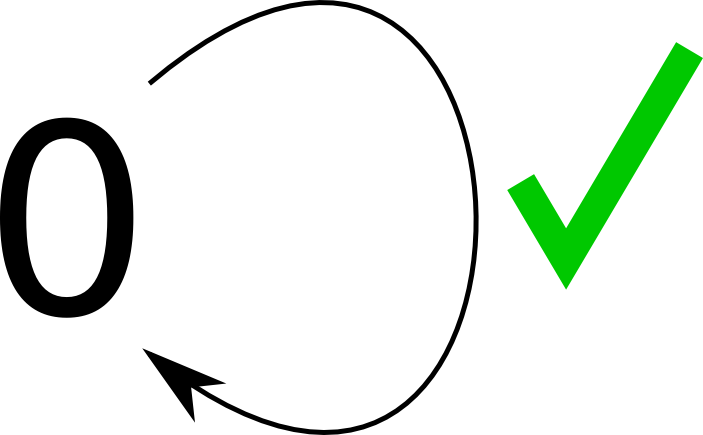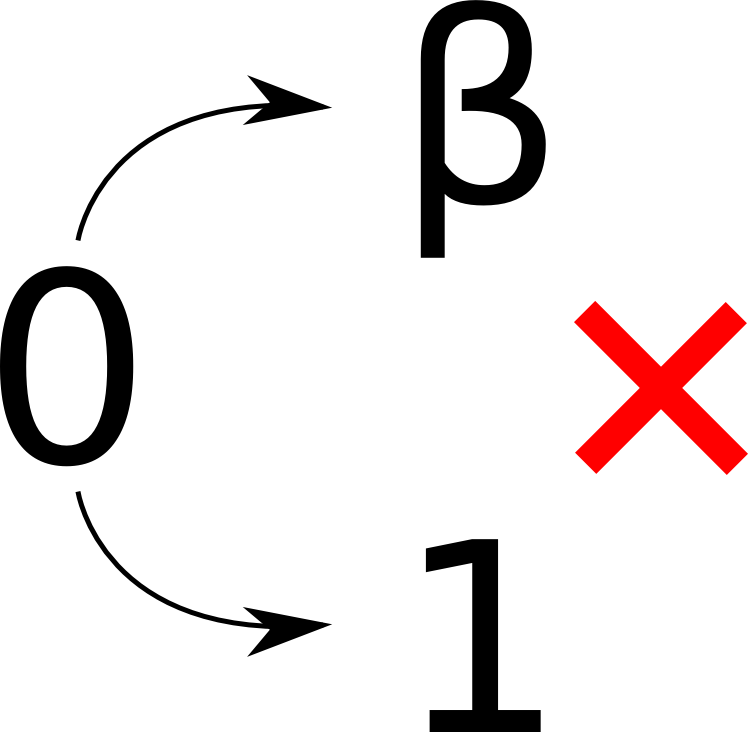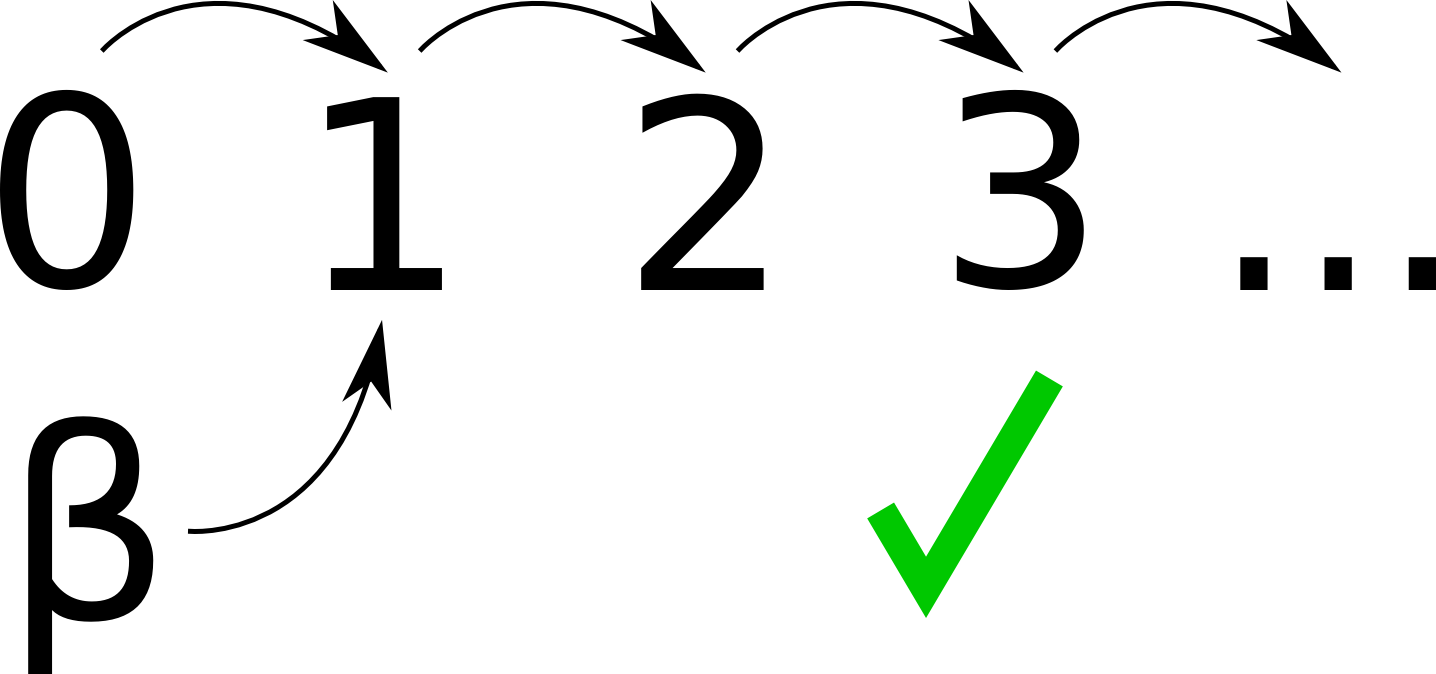# What's a number?

OK, so a bit of a silly question. Of course, we all know what numbers are. But humor me, and try to define a number without using “number”, “quantity”, or anything that refers back to a number itself.

But, before we learn numbers, we have to first understand something simpler: counting. Couldn’t we define numbers in terms of counting? Well, yes, otherwise I wouldn’t be writing this article.

Now, to not end up with a forty page book, I’m only going to go over how to define the whole numbers (I’m going to ignore negative integers, fractions, $$\pi$$, etc.)

Basically, a mathematician named Peano had a similar idea back in the 1800s. Back then, even mathematicians didn’t really know what numbers were. Some of them felt that they were simple enough that they didn’t need a definition. On the other hand, some mathematicians liked thinking about numbers in terms of geometry. Like if I draw one line, that’s 1. If I draw another line 2 times as long next to it, that’s 2, etc. It works, but it’s a bit annoying to have to work with geometry all the time. Unless you like drawing, which a lot of people go into math to avoid.

Anyway, back to Peano. During the 1800s, mathematicians were starting to ask some weirdly difficult questions. Like, how do we know that $$a + b = b + a$$? How do we even know that $$2 + 2 = 4$$? How do we even know that $$4$$ exists?

Well, we need to start somewhere, and Peano decided we needed a few basic Laws Of Mathematics for numbers (mathematicians like the word axiom). These are rules that are considered self-evident.

He came up with a total of 9. In advanced 21st century mathematics, there are generally considered to be 5. (No, it didn’t turn out that $$9 = 5$$. 4 of the axioms are really part of logic. Stuff like if $$a = b$$, $$b = a$$. In the 19th century, they didn’t really distinguish logic from math, but now we do.)

Anyway, let’s go through the Laws!

## 1. Zero is a Number

OK, so $$0$$ is a number! Very useful.

Actually, we don’t have to call this $$0$$. Let’s call it Peano.

Now Peano is a number! That’s better! Actually, no, it’s exactly the same.

All this Law Of Mathematics says is that there is some number. We haven’t really said what this number does yet. Let’s move on.

## 2. For every number $$n$$, there is a number $$n_S$$.

OK, so we know that for every number, there is another number! We have defined here basically the concept of counting. $$n_S$$ is the “next number”. Are we done?

Well, no, because I told you that there are 5 Laws Of Mathematics, and we’ve only covered 2.

Also, think about it for a second. Have we really defined counting? Does this definition tell us that we can’t, for example, have $$0_S = 0$$?No. What it does tell us, however, is that we can’t have two different $$n_S$$ for the same $$n$$.## 3. There is no number $$n$$ where $$n_S = 0$$

OK! We dealt with that annoying $$0_S = 0$$ problem! We’re done! Well, no… Try to think about a problem with this yourself before you move on.

Here it is: Let’s define $$0_S = 1$$. Now, is there anything that says that you can’t have $$\beta_S = 1$$ where $$\beta \neq 0$$?

Well, no. We have some $$0$$ (this follows Law of Mathematics #1). We have, $$0_S = 1$$, $$\beta_S = 1$$, $$1_S = 2$$ (by definition), etc. (this follows Law of Mathematics #2). We have no element $$x$$ such that $$x_S = 0$$ (this follows Law of Mathematics #3).Well, we have something that is obviously ridiculous but follows all the Laws. Well, let’s close the loophole!

## 4. For any two numbers $$x$$ and $$y$$, if $$x_S = y_S$$, $$x = y$$

OK! We’re finally done! No more weird numbers that feed into the regular numbers!

So now let’s define a few numbers:

$0_S = 1$ $1_S = 2$ $2_S = 3$ $3_S = 4$

OK, seems to be working. Now let’s define a few more.

$\gamma_S = \beta$ $\beta_S = \alpha$Where did those annoying Greek letters come from! Well, we’ve said what can be a number: the $$0, 0_S, 0_{SS}, 0_{SSS}$$ etc. And we’ve said that no number is before $$0$$. And we’ve said that no two numbers can feed into the same number.

However, what we haven’t said is that the only numbers are $$0, 0_S, 0_{SS}, 0_{SSS}$$, etc. And, guess what? That’s the fifth Law!

## 5. If something is true for $$n = 0$$ and its being true for $$n$$ makes it true for $$n_S$$, then it is true for all numbers.

OK, so this is a little more complicated than the previous examples. But let’s look at a quick example to see how it completes the definition of the numbers. $$\newcommand{\isn}{\uplus}$$

Let’s look at the symbol $$\isn$$. Basically, $$x_\isn = \true$$, or true when $$x$$ is a number, and $$\false$$, or false, whenever it is not. (I got the symbol by typing “fancy math symbols” into Google. As far as I know, it isn’t commonly used.)

Some examples:

$0_\isn = \true\ 1_\isn = \true\ 2_\isn = \true\ \spadesuit_\isn = \false\ \diamondsuit_\isn = \false$

As you can see, suits of cards are not numbers, but $$0, 1, 2$$ are.

Anyway, the first two axioms can be said as “$$0_\isn$$” and “if $$n_\isn$$, then $$(n_S)_ \isn$$”

This means that $$n_\isn$$ is true for all numbers.

Let that sink in for a minute.

OK, so basically, we proved that all numbers are numbers. But that was just to show that the fifth Law of Mathematics makes sense.

Now for a better example: let’s look at trying to get rid of those annoying Greek Letters. We defined $$\beta$$ as some number which isn’t’ the successor of anything in a chain that comes from 0. Let’s define unbetaness as $$n \neq \beta$$. We know that $$0 \neq \beta$$, and we know that if $$n \neq \beta$$ and $$n$$ is a number, $$n_S \neq \beta$$. Therefore, no number is equal to $$\beta$$, so $$\beta$$ is not a number.

Now we’re actually done! Well, not really, because we still don’t know what $$2 + 2$$ is, since we don’t know what $$+$$ means. Let’s look at the Laws of Addition.

## 1. $$0 + n = n$$

Adding nothing to something doesn’t change it. Not particularly crazy stuff.

## 2. $$a_S + b = (a + b)_ S$$

Counting up one of the numbers does the same thing as counting up the sum. In other words, if you have two piles, adding a stone to one of the piles is the same as adding a stone to the system as a whole.

# So, what is $$2 + 2$$?

$2 + 2\ 1_S + 2\ (1 + 2)_ S\ (0_S + 2)_ S\ ((0 + 2)_ S)_ S\ (2_ S)_ S\ 3_ S\ 4$

So, you’re wrong, Wikipedia, $$2 + 2$$ is not 5!

Anyway, the real point is that while before, you probably thought that $$2 + 2 = 4$$ was a basic fact, a Law of Mathematics, now you know that it is just a deduction based on a few other, simpler, laws.